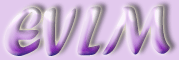Annotating graphs in MATLAB

# 1 Basic labelling

We are already familiar with producing graphs using the MATLAB "plot" command to look something like this,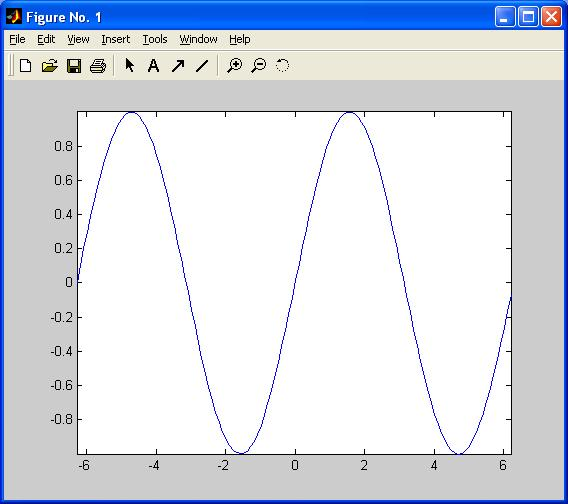using the following commands:

x=linspace(-2*pi,2*pi,500);

y=sin(x);

plot(x,y)

axis tight

Although we see that this is the graph of a sine wave, at the moment, apart from numbered tick-marks, the graph does not give us any additional information. It would be useful to add some labels indicating units, and a title. Inputting

xlabel( 'time (s)' )

ylabel( 'amplitude (mm)' )

title( 'Plot of sine wave over time period -2\pi \leq t \leq 2\pi' )

produces the following graph.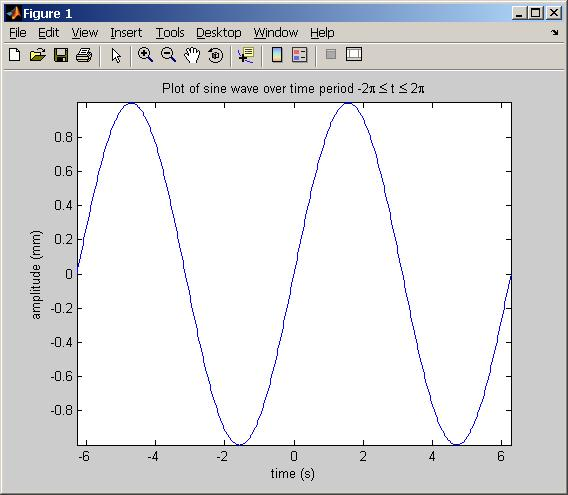Notice that the required text must be enclosed by apostrophes ('). MATLAB changes the font colour to brown to acknowledge the insertion of the first apostrophe, then to purple to acknowledge the second. If the second apostrophe is missed out, the command looks like this:

xlabel( 'time (s) )

and, if entered, gives an error output

??? xlabel('time (s))

Error: A MATLAB string constant is not terminated properly

In the title example above, the range for t is written partially in LaTeX code. MATLAB converts any text written in LaTeX to the corresponding symbol, then positions this in the text string. This enables symbols which are otherwise unavailable on the standard keyboard to be visualised.

# 2 Multiple plots

It is very simple to plot 2 or more graphs in one figure. Left click on the required figure. Now enter

hold on

y1=sin(2*x);

plot(x,y1,'r')

title( 'Plot of sine waves over time period -2\pi \leq t \leq 2\pi' )

at the command line.

The 'r' is inserted so that this line is printed in red. The last command can be easily created by pressing the $↑$ key a few times until the original command

title( 'Plot of sine wave over time period -2\pi \leq t \leq 2\pi' )

appears then left click next to "wave" and typing in the "s".

As an alternative to using the "hold on" command, it is possible to enter multiple plot commands directly into the "plot" function e.g.

plot(x,y,'b',x,y1,'r')

axis tight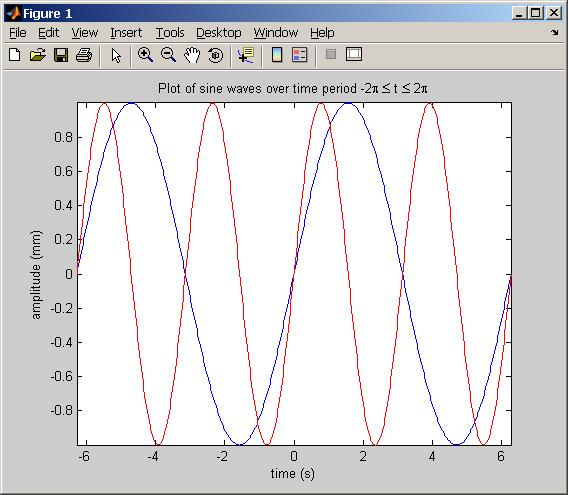We can add a legend to the graph using the following command

legend( 'sin(x)', 'sin(2x)' )

giving us the following graph, which includes the legend in the top right hand corner.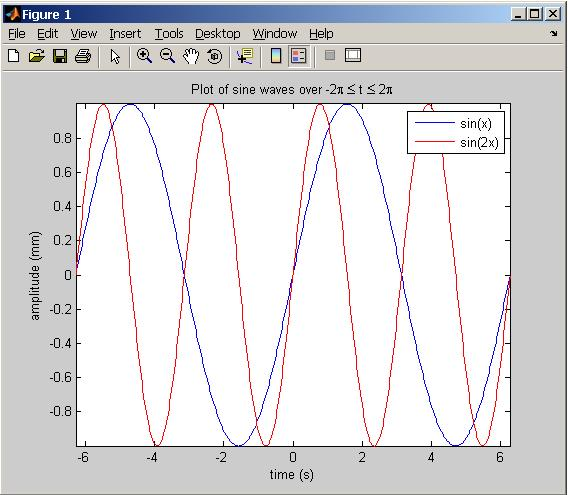The default for placement of the legend is the top right hand corner of the graph. The legend can be moved to any other position by simply left clicking on the legend and dragging it to the required place. Alternatively, by inserting a 2, 3 or 4 in the command, e.g.

legend( 'sin(x)', 'sin(2x)' , 2)

the printed position can be changed to top left, bottom left or bottom right respectively. A graph can be saved either in MATLAB as a .fig file or exported in most other formats using the "file - save as" buttons. This makes it possible to easily incorporate MATLAB figures into practically any document format.

# 3 Subplots

It is sometimes convenient to create one or more subplots in one figure, perhaps for comparison purposes.